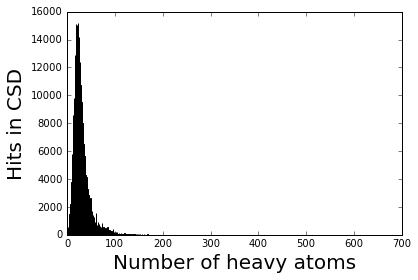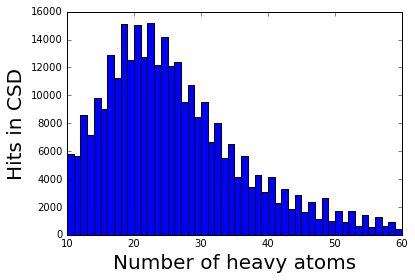Number of non-H atoms in molecules reported in the Cambridge Structural Database¶

An unexplained phenomenon in the CSD collection of molecular crystal structures is shown below.

The code below is included in Jerome Wicker’s talk from CCK-1 as a simple example of how to iterate through and extract information from the CSD using the Python API. The phenomenon has been noted many times by CCDC researchers.

The CSD Python API is used to retreive each crystal structure entry from the database using the EntryReader() iterator. The number of heavy atoms (non-hydrogen atoms) in the heaviest molecular component of organic crystal structure is appended to a list heavy_atoms.

Finally a histogram of these heavy atom counts shows that molecules with even numbers of heavy atoms are observed more frequently than those with odd numbers in the same range.

In :
%pylab inline
Populating the interactive namespace from numpy and matplotlib

This page is a copy of an interactive Python 2 notebook exported from Jupyter. In Jupyter, the %pylab inline command above loads numerical and plotting libraries and ensures that plots appear in the notebook instead of in a separate window. It isn’t required if running python from the command line, and the required libraries (numpy and matplotlib) are reimported below for convenience.

In :
from ccdc.io import EntryReader
from matplotlib import pyplot as plt
import numpy as np

heavy_atoms = []

for entry in csd_reader:
if entry.is_organic:
try:
mol = entry.molecule.heaviest_component
except:
continue
heavy_atoms.append(len(mol.heavy_atoms))

plt.figure()
plt.hist(heavy_atoms,bins=np.max(heavy_atoms))
plt.xlabel('Number of heavy atoms',fontsize=20)
plt.ylabel('Hits in CSD', fontsize=20)
plt.show()Limiting the x-axis range:

In :
plt.figure()
plt.hist(heavy_atoms,bins=np.max(heavy_atoms))
plt.xlabel('Number of heavy atoms',fontsize=20)
plt.ylabel('Hits in CSD', fontsize=20)
plt.xlim([10,60])
plt.show()In [ ]: